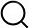# Also in the Article

2.2.5. Statistical analyses

Procedure

In the mouse models the natural variation in response to HFD feeding was used to establish correlations between plasma lipids and specified obesity endpoints as was reported in Wopereis et al . Correlations and corresponding p-values were calculated in R version 3.6.3 using Spearman's tests. The resulting p-values were corrected for multiple testing by calculating the false discovery rate (FDR) using the Benjamini Hochberg method. Graphs were produced using GraphPad Prism version 8.4.2.

Infancy age- and sex-appropriate standard deviation scores (SDS) were calculated for weight and length measurements, (with adjustment for gestational age at birth and 3 months), by comparison to the UK 1990 growth reference using LMSgrowth software . Internally-derived SDS were calculated for each individual skinfolds, adjusted for infant sex, GA, and exact age at visit. Growth gains were derived from delta weight, height, and mean skinfolds SDS between 3-12 and 12-24 months. Maternal BMI was derived from self-reported pre-pregnancy weight divided by the square of measured height (kg/m2).

Unless otherwise stated, all descriptive data are presented as means±standard deviations (SD) for continuous variables or as a percentage (%) for categorical variables. Multiple linear regression was used to investigate associations between lipid ratios separately at 3 months of age with weight, height, and skinfolds (reflecting adiposity) gains during both 3-12 and 12-24 months. For weight and height SDS, models were adjusted for maternal parity, maternal pre-pregnancy BMI, and infant feeding history. For mean skinfold, models were adjusted for infant sex, gestational age, postnatal age at measurement, and feeding history, as well as maternal pre-pregnancy BMI and parity. For linear regression models, assumptions of normality, linearity, homoscedasticity, and absence of multicollinearity had been evaluated and none were violated. Since there were only 6 variables/lipid ratios in the regression models, statistical significance was achieved if p values<0.05. Statistical analyses were carried out using SPSS version 25.0 (IBM) and R version 1.0.136.

Q&A